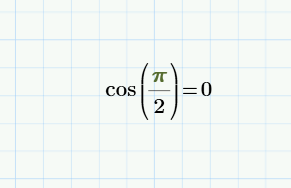cancel
Showing results for
Did you mean:
cancel
Showing results for
Did you mean:

Community Tip - You can Bookmark boards, posts or articles that you'd like to access again easily! X

## cos(pi/2) does not equal 0...why?1-Newbie

## cos(pi/2) does not equal 0...why?

I am performing some martrix algebra.

When I ask Mathcad what cos(pi/2) equals it says "6.123X10^-17". How do I get this value to revert to 0, which is the true value?

Thank you

Jamie

9 REPLIES 91-Newbie
(To:ptc-6668487)

I was able to change the formatting from general to decimal which fixed the formatting of zero; however my large numbers now have 5-6 zeroes beyond the significant digits.

Is there a way to get 73680000 to show as 7.37X10^7 and zero to show as 0, not 2.8X10^-9?

Thank you24-Ruby V
(To:ptc-6668487)
 Jamie Skovron wrote:I was able to change the formatting from general to decimal which fixed the formatting of zero; however my large numbers now have 5-6 zeroes beyond the significant digits.Is there a way to get 73680000 to show as 7.37X10^7 and zero to show as 0, not 2.8X10^-9?

Change formatting back to General or Scientific. You may play araound with the value of the Zero Threshold (by default 15, you may try to lower it to 8).

If the calculated value IS 2.8X10^-9 I would like it to show up as 2.8X10^-9 and not as zero.

rather recheck the ways you do your calculations and see if you can achieve a better precision without big roundoff errors.

And in Mathcad cos(pi/2) definitely is shown as 0 and not anything else.

You'll have to show your worksheet for further help.1-Newbie
(To:Werner_E)As you can see, it is calculating cos^2-sin^2 as 2.22X10^-16 rather than zero.24-Ruby V
(To:ptc-6668487)

I see, thats normal roundoff errors.

Its easy to suppress that in Mathcad (15 and below) - in fact you won't see anything else than 0 there in the first place anyway.

But I fear there is no way to do what you want with the limited formatting capabilities of Prime other than using symbolic evaluation.19-Tanzanite
(To:Werner_E)

I wrote this years ago, and it works in MC15 (although I forget now why I wrote it). It works in Prime, but only if you turn off Approximate Equality under the calculation options, and that can lead to other problems.17-Peridot
(To:ptc-6668487)Set Math Formatting to Decimal15-Moonstone
(To:ptc-6668487)

I met the same in calculations of attack angle of wind on elements of my structure. I was very surprised.

So, as I understood I have to round my velocity vectors to get right angles.23-Emerald III
(To:ifomenko)

You have to realize that (unless you work fully symbolically) Mathcad uses numerical approximation. So you may get results close to, but not exactly, zero on occasions.  cos(pi/2) is one such occasion:In (real) Mathcad, it is possible to set the zero threshold, which will cause numerical resuls to show as 0 when they are close to zero within that threshold. The default for the zero threshold is '15' which sets that any value within -10^-15 to 10^-15 will be shown as zero. 'Shown as'....! it will not be set to 0. If you multiply such a result with 10^15, it will come up as non-zero. See here:So what you could do is wrap the cos function into a function that detects small results, and sets them to 0. Like:Success!

Luc15-Moonstone
(To:LucMeekes)

Thanks, I used round(cos(),8)Announcements